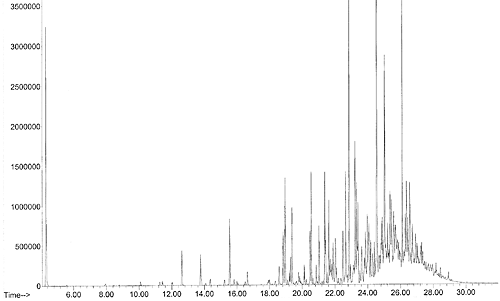# USGS Method-I-4724A database containing more than 16,300 discharge values and ancillary hydraulic attributes was assembled from summaries of discharge measurement records for 391 USGS streamflow-gauging stations (streamgauges) in Texas. Each discharge is between the 40th- and 60th-percentile daily mean streamflow as determined by period-of-record, streamgauge-specific, flow-duration curves. Each discharge therefore is assumed to represent a discharge measurement made for near-median streamflow conditions, and such conditions are conceptualized as representative of midrange to baseflow conditions in much of the state.

The hydraulic attributes of each discharge measurement included concomitant cross-section flow area, water-surface top width, and reported mean velocity. Two regression equations are presented: (1) an expression for discharge and (2) an expression for mean velocity, both as functions of selected hydraulic attributes and watershed characteristics. Specifically, the discharge equation uses cross-sectional area, water-surface top width, contributing drainage area of the watershed, and mean annual precipitation of the location; the equation has an adjusted R-squared of approximately 0.95 and residual standard error of approximately 0.23 base-10 logarithm (cubic meters per second).

The mean velocity equation uses discharge, water-surface top width, contributing drainage area, and mean annual precipitation; the equation has an adjusted R-squared of approximately 0.50 and residual standard error of approximately 0.087 third root (meters per second).There are no products listed under this category.